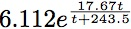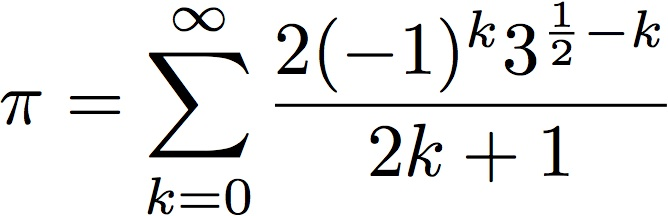# Homework #1

 Due: Friday, October 12, 2012 at 5:00PM Points: 100

Please turn in your answers for the homework assignment on SmartSite, under Homework #1 in Assignments. Turn in your programs answers for the extra credit under Extra Credit #1 there.

1. (30 points) Write a program that asks the user for the temperature t in degrees Celsius and then displays the estimated vapor pressure of water vapor in millibars at that temperature using the approximationUse a function to compute the approximation. Your program should print the output in exactly this form (you may change the number of digits after the decimal point, but you must have at least two):

```Enter temperature in degrees C: 10↵
At this temperature, the vapor pressure is approximately 12.2716959939 millibars
```

Here, what the computer prints is in regular font, what you type is in italic font, and the symbol “↵” represents a return or enter. Use your program to estimate the temperature at which the vapor pressure is approximately 10 millibars (to two decimal places).

Call this program “vp.py”, and put your answer in a comment at the beginning of the program.

2. (40 points) Modify the program you wrote for question 1 to print the estimated vapor pressures for temperatures between −20 and 50 degrees Celsius at 5 degree intervals. Your output should look like this:
```temp	  pressure
----      --------
-20	    1.26
…
50	  124.02
```
with the proper numbers substituted for the “…”s (so you need only compute the vapor pressure to 2 decimal places). Call this program “vptable.py”.

Hint: One way to print the table is to use the format string “"%3d\t%6.2f" % (i , vp(i))”, where i is the temperature and vp(i) is the function to compute the vapor pressure from the temperature i.

3. (40 points) The goal of the following program is to convert a temperature from degrees Farenheit to degrees Celsius. The formula is:where F is the number of degrees Fahrenheit and C the number of degrees Celsius.

Here is the program:

```ftemp = input("Enter degrees in Fahrenheit: ")
ctemp = 5 // 9 * (ftemp - 32)
print(ftemp, "degrees Fahrenheit is", ctemp, "degrees centigrade")
```

But there are two problems:

1. Whenever I run the program, it gives me a TypeError message as soon as I enter my number.
2. Once I fixed this problem, it always tells me the result is −0.0 degrees Celsius.

Please fix both these problems, so the program converts Fahrenheit to Celsius correctly. The program must handle floating point numbers, so entering “32.5” should produce a (small) real number, not a ValueError.

Call your fixed program “ftoc.py”, and explain what caused the two problems in a comment at the beginning of the program.

## Extra Credit

Remember to hand this in under Extra Credit #1, not under Homework #1!

Abraham Sharp developed an infinite sum that produces π:1. (20 points) Compute and print the resulting approximations to π for the first 5, 10, …, 50 terms. Each line of your output should look like this:
```After 5 terms, the approximation is 3.1426047456630846
```
Hint: Your number may differ; this is intended to show you the format of the output only.
2. (10 points) After what term does adding extra terms stop improving the approximation?

Call your program ``sharp.py'', and put your answer to the second question in a comment at the beginning of the program.ECS 10, Basic Concepts of Computing Fall Quarter 2012